# Dual polyhedron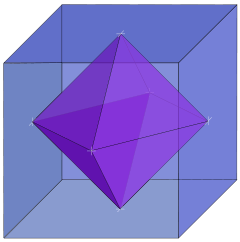The dual of a cube is an octahedron, shown here with vertices at the cube face centers.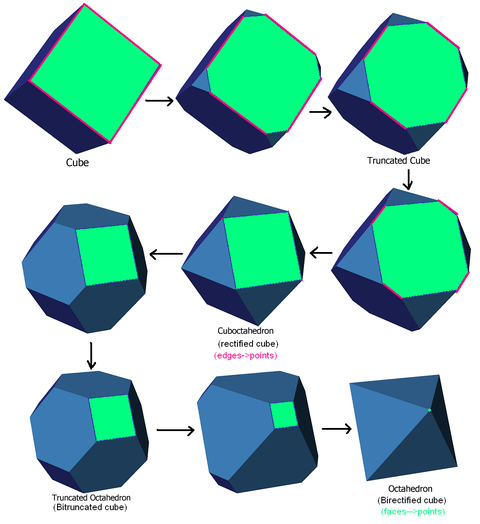Truncation sequence from a cube to its dual octahedron. A polyhedral dual is called a face-rectification or a birectification.

In geometry, polyhedra are associated into pairs called duals, where the vertices of one correspond to the faces of the other. Starting with any given polyhedron, the dual of its dual is the original polyhedron. The dual of an isogonal polyhedron, having equivalent vertices, is one which is isohedral, having equivalent faces; the dual of an isotoxal polyhedron (having equivalent edges) is also isotoxal. The regular polyhedra  the Platonic solids and Kepler-Poinsot polyhedra  form dual pairs, with the exception of the regular tetrahedron which is self-dual.

Duality is closely related to reciprocity or polarity.

## Kinds of duality

There are many kinds of duality. The kinds most relevant to elementary polyhedra are:

• polar reciprocity
• topological or abstract duality

### Polar reciprocation

The duality of polyhedra is most commonly defined in terms of polar reciprocation about a concentric sphere. Here, each vertex (pole) is associated with a face plane (polar plane or just polar) so that the ray from the center to the vertex is perpendicular to the plane, and the product of the distances from the center to each is equal to the square of the radius. In coordinates, for reciprocation about the spherethe vertexis associated with the plane.

The vertices of the dual are the poles reciprocal to the face planes of the original, and the faces of the dual lie in the polars reciprocal to the vertices of the original. Also, any two adjacent vertices define an edge, and these will reciprocate to two adjacent faces which intersect to define an edge of the dual. This dual pair of edges are always orthogonal (at right angles) to each other.

Ifis the radius of the sphere, andandrespectively the distances from its centre to the pole and its polar, then:For the more symmetrical polyhedra having an obvious centroid, it is common to make the polyhedron and sphere concentric, as in the Dorman Luke construction described below.

However, it is possible to reciprocate a polyhedron about any sphere, and the resulting form of the dual will depend on the sphere chosen; as we move the sphere around, the dual form distorts. The choice of center (of the sphere) is sufficient to define the dual up to similarity. If multiple symmetry axes are present, they will necessarily intersect at a single point, and this is usually taken to be the centroid. Failing that, a circumscribed sphere, inscribed sphere, or midsphere (one with all edges as tangents) can be used.

If a polyhedron has an element passing through the center of the sphere, the corresponding element of its dual will go to infinity. Since traditional "Euclidean" space never reaches infinity, the projective equivalent, called extended Euclidean space, must be formed by adding the required 'plane at infinity'. Some theorists prefer to stick to Euclidean space and say that there is no dual. Meanwhile, Wenninger (1983) found a way to represent these infinite duals, in a manner suitable for making models (of some finite portion!).

The concept of duality here is closely related to the duality in projective geometry, where lines and edges are interchanged; in fact it is often mistakenly taken to be a particular version of the same. Projective polarity works well enough for convex polyhedra. But for non-convex figures such as star polyhedra, when we seek to rigorously define this form of polyhedral duality in terms of projective polarity, various problems appear. See for example Grünbaum & Shepherd (1988), and Gailiunas & Sharp (2005). Wenninger (1983) also discusses some issues on the way to deriving his infinite duals.

#### Canonical duals

Any convex polyhedron can be distorted into a canonical form, in which a midsphere (or intersphere) exists tangent to every edge, such that the average position of these points is the center of the sphere, and this form is unique up to congruences.

If we reciprocate such a polyhedron about its intersphere, the dual polyhedron will share the same edge-tangency points and so must also be canonical; it is the canonical dual, and the two together form a canonical dual pair.

### Topological duality

We can distort a dual polyhedron such that it can no longer be obtained by reciprocating the original in any sphere; in this case we can say that the two polyhedra are still topologically or abstractly dual.

The vertices and edges of a convex polyhedron can be projected to form a graph (sometimes called a Schlegel diagram) on the sphere or on a flat plane, and the corresponding graph formed by the dual of this polyhedron is its dual graph.

An abstract polyhedron is a certain kind of partially ordered set (poset) of elements, such that adjacencies, or connections, between elements of the set correspond to adjacencies between elements (faces, edges, etc.) of a polyhedron. Such a poset may be "realised" as a geometrical polyhedron having the same topological structure. The poset may be represented in a Hasse diagram. Any such poset has a dual poset. The Hasse diagram of the dual polyhedron is obtained very simply, by reading the original diagram upside-down.

## Dorman Luke construction

For a uniform polyhedron, the face of the dual polyhedron may be found from the original polyhedron's vertex figure using the Dorman Luke construction. This construction was originally described by Cundy & Rollett (1961) and later generalised by Wenninger (1983).

As an example, here is the vertex figure (red) of the cuboctahedron being used to derive a face (blue) of the rhombic dodecahedron.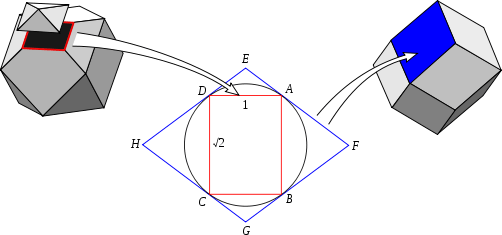Before beginning the construction, the vertex figure ABCD is obtained by cutting each connected edge at (in this case) its midpoint.

Dorman Luke's construction then proceeds:

1. Draw the vertex figure ABCD
2. Draw the circumcircle (tangent to every corner A, B, C and D).
3. Draw lines tangent to the circumcircle at each corner A, B, C, D.
4. Mark the points E, F, G, H, where each tangent line meets the adjacent tangent.
5. The polygon EFGH is a face of the dual polyhedron.

In this example the size of the vertex figure was chosen so that its circumcircle lies on the intersphere of the cuboctahedron, which also becomes the intersphere of the dual rhombic dodecahedron.

Dorman Luke's construction can only be used where a polyhedron has such an intersphere and the vertex figure is cyclic, i.e. for uniform polyhedra.

## Self-dual polyhedra

Topologically, a self-dual polyhedron is one whose dual has exactly the same connectivity between vertices, edges and faces. Abstractly, their Hasse diagrams are identical.

A geometrically self-dual polyhedron is not only topologically self-dual, but its polar reciprocal about some given point, typically its centroid, is a congruent figure. For example, the dual of a regular tetrahedron is another regular tetrahedron, (reflected through the origin).

Every polygon is topologically self-dual (it has the same number of vertices as edges, and these are switched by duality), but will not in general be geometrically self-dual (up to rigid motion, for instance). Regular polygons are geometrically self-dual: all angles are congruent, as are all edges, so under duality these congruences swap).

The most common geometric arrangement is where some convex polyhedron is in its canonical form, which is to say that all its edges must be tangent to a certain sphere whose centre coincides with the centre of gravity (average position) of the tangent points. If the figure is self-dual, then the polar reciprocal is congruent to it.

There are infinitely many geometrically self-dual polyhedra. The simplest infinite family are the pyramids of n sides and of canonical form. Another infinite family, elongated pyramids, consists of polyhedra that can be roughly described as a pyramid sitting on top of a prism (with the same number of sides). Add a frustum (pyramid with the top cut off) below the prism and you get another infinite family, and so on.

There are many other convex, self-dual polyhedra. For example, there are 6 different ones with 7 vertices, and 16 with 8 vertices.

Non-convex self-dual polyhedra can also be found, such as the excavated dodecahedron.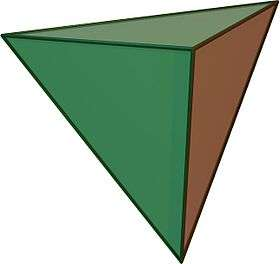3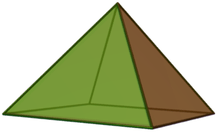4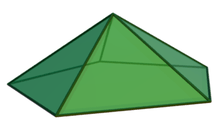5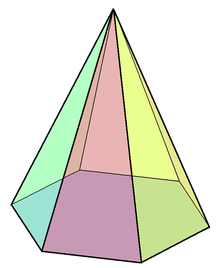6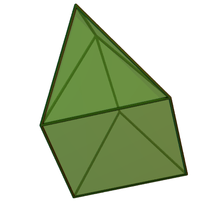3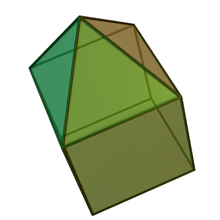4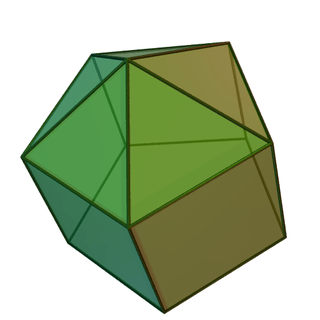5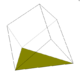3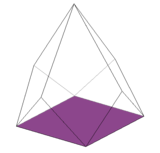45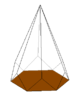6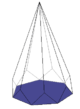7

### Self-dual compound polyhedra

Trivially, the compound of any polyhedron and its dual is a self-dual figure.

If a polyhedron is self-dual, then the compound of the polyhedron with its dual will comprise congruent polyhedra. The regular compound of two tetrahedra, known as the Stella octangula, is the only regular compound with this property.

## Dual polytopes and tessellations

Duality can be generalized to n-dimensional space and dual polytopes; in two dimension these are called dual polygons.

The vertices of one polytope correspond to the (n 1)-dimensional elements, or facets, of the other, and the j points that define a (j 1)-dimensional element will correspond to j hyperplanes that intersect to give a (n j)-dimensional element. The dual of an n-dimensional tessellation or honeycomb can be defined similarly.

In general, the facets of a polytope's dual will be the topological duals of the polytope's vertex figures. For regular and uniform polytopes, the dual facets will be the polar reciprocals of the original's vertex figure. For example, in four dimensions, the vertex figure of the 600-cell is the icosahedron; the dual of the 600-cell is the 120-cell, whose facets are dodecahedra, which are the dual of the icosahedron.

### Self-dual polytopes and tessellations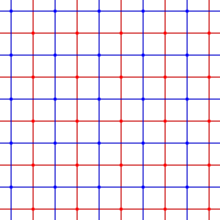The square tiling, {4,4}, is self-dual, as shown by these red and blue tilings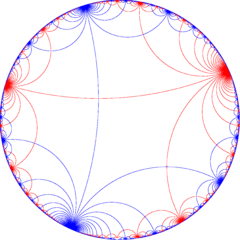The Infinite-order apeirogonal tiling, {,} in red, and its dual position in blue

The primary class of self-dual polytopes are regular polytopes with palindromic Schläfli symbols. All regular polygons, {a} are self-dual, polyhedra of the form {a,a}, 4-polytopes of the form {a,b,a}, 5-polytopes of the form {a,b,b,a}, etc.

The self-dual regular polytopes are:

The self-dual (infinite) regular Euclidean honeycombs are:

The self-dual (infinite) regular hyperbolic honeycombs are:Wikimedia Commons has media related to Dual polyhedron.